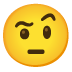# How to get a turnkey!

I’m going to adjust the angle of the cannon
with the orientation key on the keyboard.
How can I get a orientation key?usually the WASD or the UPDOWNLEFTRIGHT keyboard keys are used.
and set a X/Y position.
here a version what show both ( 2 objects // even 2 player )

``````int p1x,p1y,p2x,p2y;

void setup(){}
void draw(){}

void keyPressed() { //__ we check on KEY operation
if      (keyCode == UP    )   p1y--;
else if (key == 'w'       )   p2y--;
else if (keyCode == DOWN  )   p1y++;
else if (key == 's'       )   p2y++;
else if (keyCode == RIGHT )   p1x++;
else if (key == 'd'       )   p2x++;
else if (keyCode == LEFT  )   p1x--;
else if (key == 'a'       )   p2x--;
println("after move: 1 ", p1x, ",", p1y, "/ 2 ", p2x, ",", p2y);
} // end keyPressed

``````
1 Like